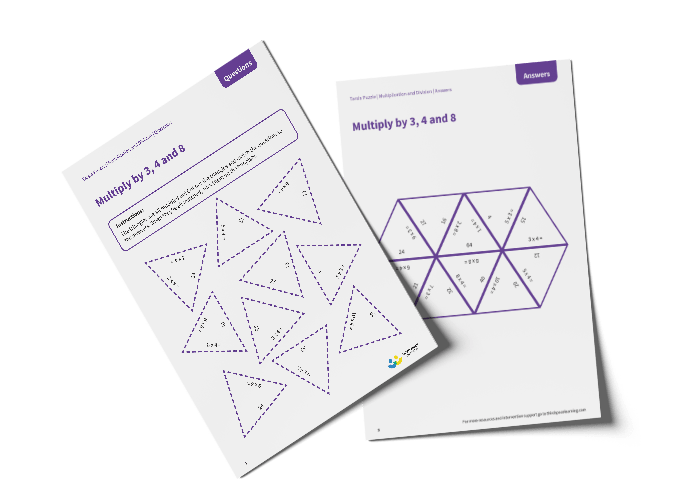# Tarsia Puzzle Multiply by 3, 4 and 8 (Year 3)

The triangles in the tarsia puzzle have all been mixed up. In this multiplying by 3, 4 and 8 tarsia puzzle, pupils will need to match the multiplication question to the correct answer to create one large hexagon.

This Year 3 multiply by 3, 4 and 8 tarsia puzzle worksheet contains:

• One question sheet
• One template sheet

Year 3

Multiplication